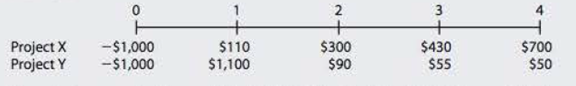Chapter 11, Problem 13P### Fundamentals of Financial Manageme...

9th Edition
Eugene F. Brigham + 1 other
ISBN: 9781305635937

#### Solutions

Chapter
Section### Fundamentals of Financial Manageme...

9th Edition
Eugene F. Brigham + 1 other
ISBN: 9781305635937
Textbook Problem
4 views

# MIRR A firm is considering two mutually exclusive projects, X and Y, with the following cash flows:The projects are equally risky, and their WACC is 11%. What is the MIRR of the project that maximizes shareholder value?

Summary Introduction

To calculate: The MIRR of the project which maximizes shareholders’ wealth.

Introduction:

Net Present Value (NPV):

It is a method under capital budgeting which includes the calculation of net present value of the project in which a company is investing. The calculation is done by calculating the difference between the value of cash inflow and value of cash outflow after considering the discounted rate.

Modified Internal Rate of Return (MIRR):

It refers to the rate of return that is computed by the company to make a decision regarding the selection and ranking of a project for investment. This is a modified version of IRR with reinvestment of cash flows at the cost of capital.

Explanation

The calculation of NPV, IRR and MIRR of Project X on spreadsheet is:

Table (1)

The NPV of the Project X is \$118.11 and MIRR is 14.14%.

The calculation of NPV, IRR and MIRR of Project Y on spreadsheet is:

### Still sussing out bartleby?

Check out a sample textbook solution.

See a sample solution

#### The Solution to Your Study Problems

Bartleby provides explanations to thousands of textbook problems written by our experts, many with advanced degrees!

Get Started

#### Find more solutions based on key concepts